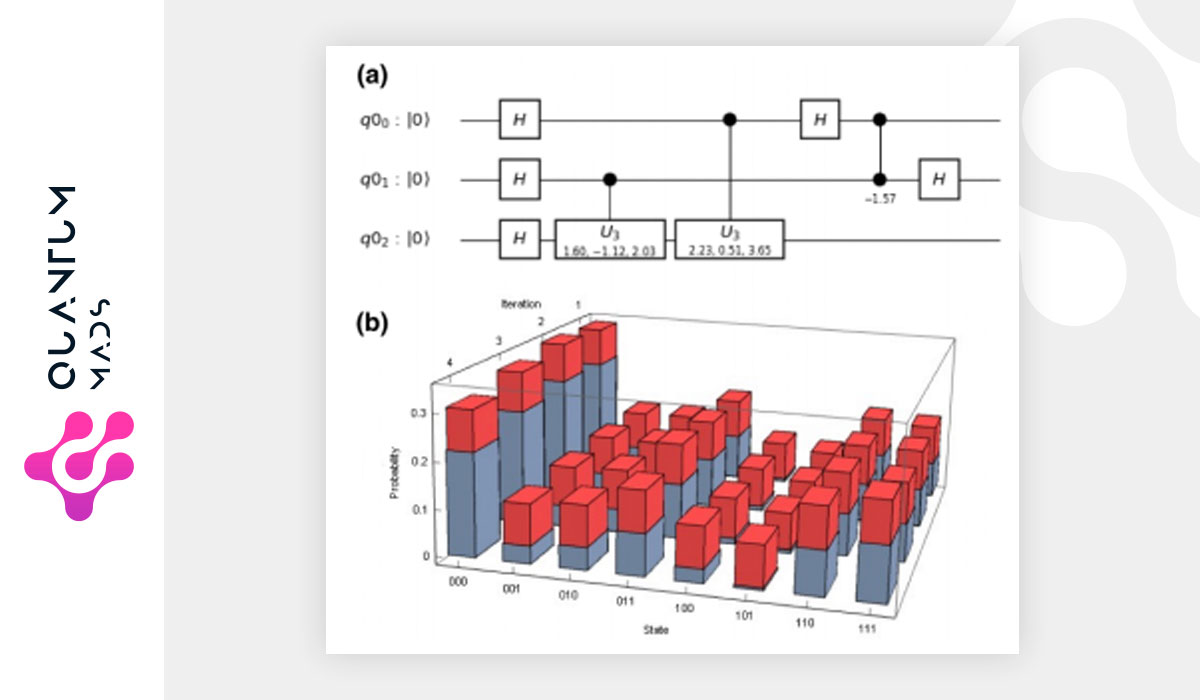Saltear al contenido principalPricing interest-rate financial derivatives is a major problem in finance, in which it is crucial to accurately reproduce the time-evolution of interest rates. Several stochastic dynamics have been proposed in the literature to model either the instantaneous interest rate or the instantaneous forward rate. A successful approach to model the latter is the celebrated Heath-Jarrow-Morton framework, in which its dynamics is entirely specified by volatility factors.

On its multifactor version, this model considers several noisy components to capture at best the dynamics of several time-maturing forward rates. However, as no general analytical solution is available, there is a trade-off between the number of noisy factors considered and the computational time to perform a numerical simulation. Here, we employ the quantum principal component analysis to reduce the number of noisy factors required to accurately simulate the time evolution of several time-maturing forward rates.

The principal components are experimentally estimated with the 5-qubit IBMQX2 quantum computer for 2×2 and 3×3 cross-correlation matrices, which are based on historical data for two and three time-maturing forward rates. This manuscript is a first step towards the design of a general quantum algorithm to fully simulate on quantum computers the Heath-Jarrow-Morton model for pricing interest-rate financial derivatives. It shows indeed that practical applications of quantum computers in finance will be achievable in the near future.

Read the whole article on arXiv

Volver arriba